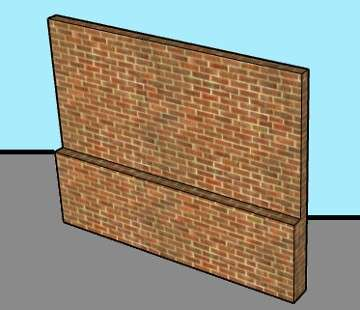# Compound Wall’s Bricks, Cement, and Sand Estimator

## Compound wall Materials (Bricks, Mortar) Calculator## How to Estimate No. of Bricks, Cement, and Sand in Compound Wall?

Q) Calculate the Quantity of Bricks, Cement, Sand in a compound wall having Length = 30 feet, Breadth = 20 feet,

Height above the floor of wall = 5 feet with 5” width.

Height below ground = 3 feet with 10” width.

Bricks Size:- 190x90x90 mm

Step1:- Calculate volume of Wall

Above Ground = L x B x H = 100 x 5/12 x 5

= 208.3 Cub. Feet

Below Ground = 100 x 10/12 x 3 = 250 Cub. Feet

Total Volume of Wall = 458.3 Cub. Feet = 12.98 cub. meter

Step2:- Calculate volume of Bricks without Mortar

V.B = L x B x H = 0.190 x 0.09 x 0.09 = 0.001539 cub. Meter

Assume 10 mm thickness of mortar

Step3:- Calculate volume of Bricks with Mortar

V.B = L x B x H = 0.200 x 0.100 x 0.100 = 0.002 cub. meter

Step4:- Calculate no. of bricks

Divide volume of wall by volume of bricks with mortar

N.B = 12.98/0.002 = 6490 Nos.

Step5:- Calculate Wet volume Mortar

V.M = Volume of the wall – No. of Bricks x Volume of one brick without mortar

= 13 – 6500 x 0.001539 = 2.99 = 3 cubic meter Approx.

Step6 :- Calculate Dry volume of the Mortar

Dry Volume of mortar x 1.34 ( Add 32% to 34%)

= 2.99 x 1.34 = 4 cubic meter

Assume ratio of (Cement : Sand) = (1:4)

Step7:- Calculate the volume of Sand and Cement

V.S = Sand Ratio /(1+4) x Dry Volume

= 4/5 x 4 = 3.2 cub. Meter

V.C = Cement Ratio /(1+4) x Dry Volume

= 1/5 x 4 = 0.8 cub. Meter

Weight of cement = Volume x density of cement = 0.8 x 1440 = 1152 Kg.

No. of Bags of Cement = 1152/50 = 23.04 Bags of Cement ( One Bags of cement = 50 Kg.)

Summary,